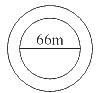# Ex.11.3 Q13 Perimeter and Area - NCERT Maths Class 7

Go back to  'Ex.11.3'

## Question

A circular flower bed is surrounded by a path $$4 \rm\,m$$ wide. The diameter of the flower bed is $$66 \rm\,m$$. What is the area of this path? $$(π = 3.14)$$Video Solution
Perimeter And Area
Ex 11.3 | Question 13

## Text Solution

What is known?

A circular flower bed of diameter $$66 \rm\,m$$ which is surrounded by a path $$4 \rm\,m$$ wide.

What is unknown?

The area of the path.

Reasoning:

Since the diameter of the circular flower bed is $$66\rm\,m,$$ so, the radius of the circular bed will be $$33\rm\,m$$. The radius of the outer circle, which includes radius of the circular path as well width of the path is $$33\rm\,m + 4\rm\,m = 37\rm\,m$$. Now the areas of the inner circle of radius $$33\rm\,m$$ and outer circle of radius $$37 \rm\,m$$ can be calculated and by subtracting area of the inner circle from the area of the outer circle you will get the area of this path.

Steps:

Diameter of the circular flower bed $$=$$ $$66 \rm\,m$$

$$\therefore$$ Radius of circular flower bed (r) $$=$$ \begin{align}\frac{{66}}{2}\end{align} $$= 33 \rm\,m$$

$$\therefore$$ Radius of circular flower bed with $$4 \rm\,m$$ wide path $$(R) = 33 + 4 = 37 \rm\,m$$

Area of path = Area of bigger circle-Area of smaller circle

\begin{align}&=\pi {{\rm{R}}^2} - \pi {r^2}\\&= \pi \left( {{{\rm{R}}^2} - {r^2}} \right)\\ &= 3.14\left[ {{{\left( {37} \right)}^2} - {{\left( {33} \right)}^2}} \right]\\ &= 3.14\left [{\left({37 + 33} \right)\left( {37-33} \right)} \right] \end{align}

Using this formula,

\begin{align} (a^2 -b^2)= [( {a + b} ) ( {a - b} )]\end{align}

\begin{align} & = 3.14 \times 70 \times 4\\ &= 879.20\,\rm {m^2}\end{align}

Therefore, the area of the path is $$879.20 \rm\,m^2.$$

Learn from the best math teachers and top your exams

• Live one on one classroom and doubt clearing
• Practice worksheets in and after class for conceptual clarity
• Personalized curriculum to keep up with school